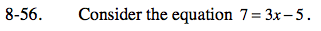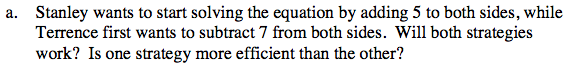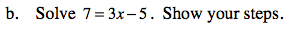Home > ACC7 > Chapter cc38 > Lesson cc38.2.1 > Problem8-56

8-56.
1. Consider the equation 7 = 3x − 5. Homework Help ✎

1. Stanley wants to start solving the equation by adding 5 to both sides, while Terrence first wants to subtract 7 from both sides. Will both strategies work? Is one strategy more efficient than the other?

2. Solve 7 = 3x − 5. Show your steps.Solving the equation ultimately involves isolating x to determine its value. If both strategies are working towards isolating x through different methods, will both strategies work?

Does one strategy require fewer steps to isolate x? Is the strategy of adding 5 to both sides rather than subtracting 7 a more efficient and direct method?7 = 3x − 5

Add 5 to both sides.

12 = 3x

4 = x